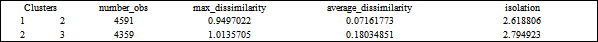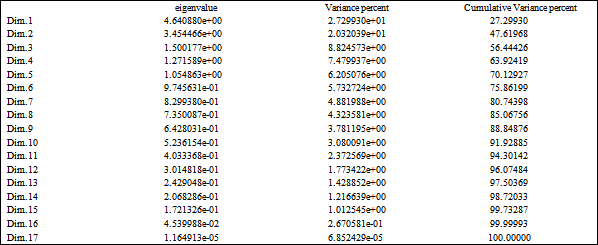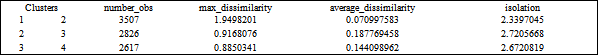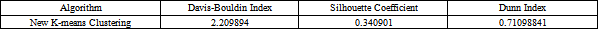## Tables index

#### Incorporating K-means, Hierarchical Clustering and PCA in Customer Segmentation

Journal of City and Development. 2021, 3(1), 12-30• Table 1. basic statistics of the clusters resulted from the Hierarchical clustering• Table 2. basic statistics of the clusters resulted from applying the K-means• Table 3. different validation metrics for the Hierarchical and K-means clustering• Table 4. The importance of initial principal components Importance of components• Table 5. the eigen values and variance% of the initial PCs (dimensions)• Table 6. data frame of the 5 optimal PCs• Table 7. the variance explained by the optimal PCs• Table 8. basic statistics for the clusters resulted from the PCA• Table 9. validation metrics for the updated K-means clustering